Next: Unitary operators Up: Bra-ket notation Previous: Projection operators

### Matrix representation of operators

Above we suggested that operators in Hilbert space can be written as a sum of outer products. However, it is much easier to work with operators that are expressed as matrices. Given operator Y and a basis set of kets, we can construct antable of complex numbers whose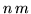th element is given by <an| Y | am>. The set of n2 complex numbers can most conveniently be written as a square matrix, where m is a column index and n a row index. We use the notation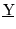to represent this matrix, and theth element of that matrix is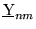. The matrixis called the matrix representation of operator Y. Show that we can express Y in the form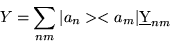(1.39)

Consider the following equation in ket space,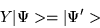(1.40)

where Y is an operator and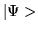an arbitrary ket. We take the inner product of this equation with state | am>(Remember, taking an inner product of two kets involves multiplying on the left by a bra), or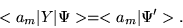(1.41)

Evaluating this expression we obtain,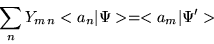(1.42)

which can be written as a matrix equation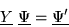(1.43)

where we defined a column matrix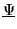whose n'th row has the entry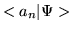. The utility of a matrix representation for operator Y and ketis now apparent. We can replace any abstract operator equation, such as Eq. (17), with a more familiar matrix equation (20).

We can do the same in bra space. For example, consider the adjoint of Eq. (17)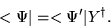(1.44)

Show that the matrix representation of this equation is given by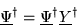(1.45)

where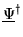is the adjoint matrix of the column matrix(note: you can consider the adjoint of a column matrix to be a row matrix). Note that the n m 'th element of matrix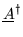is the complex conjugate of the m n'th element of matrix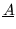, i.e.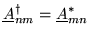Next: Unitary operators Up: Bra-ket notation Previous: Projection operators
Bernard Zygelman
1999-09-21